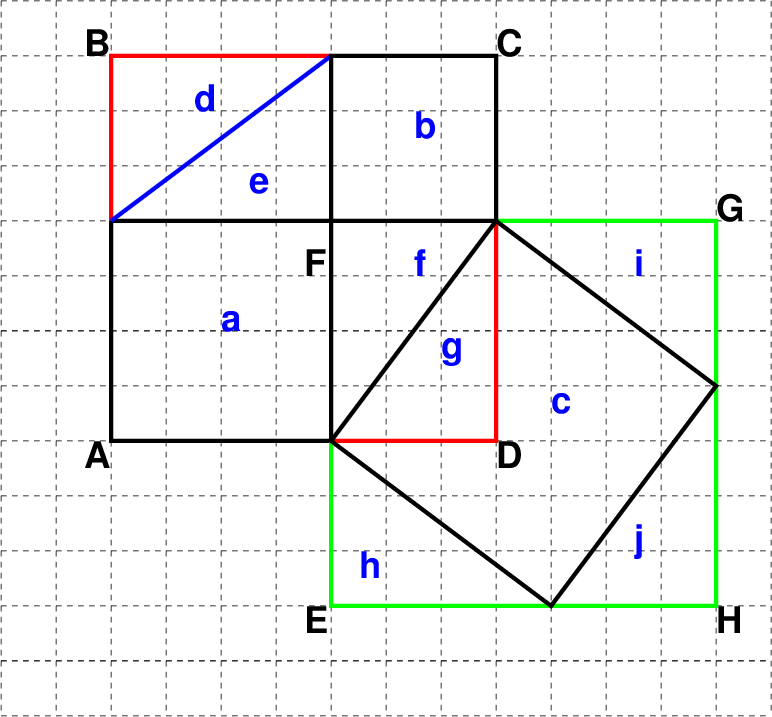## Pythagorean Theorem Proof

version = 1.03 of pythagoras.tex 2012 Jun 14

https://alum.mit.edu/www/toms/

Prove the Pythagorean theorem myself with no help. Do it from scratch geometrically.Equal Areas: triangles d, e, f, g, h, i, j T since they are all 3 × 4.
Any other side sizes also work.

Equal Areas: squares ABCD and EFGH since they are both (3 + 4) × (3 + 4) = 7 × 7

But ABCD = a + b + d + e + f + g = a + b + 4T

and EFGH = c + f + h + i + j = c + 4T

So the areas of the three squares a, b and c are related by:

a + b = c

QED!!!
_________________________________________________________________________________________

Sketch: xfig (http://www.xfig.org/)
document: latex (https://alum.mit.edu/www/toms/latex.html)
convert to html: tex4ht (http://tug.org/applications/tex4ht/mn.html)